# Abelian integral

(diff) ← Older revision | Latest revision (diff) | Newer revision → (diff)

algebraic integral

An integral of an algebraic function, i.e. an integral of the form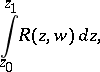(1)

whereis some rational function in variablesthat are related by an algebraic equation(2)

with coefficients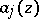that are polynomials in,. To equation (2) there corresponds a compact Riemann surfacewhich is an-sheeted covering of the Riemann sphere. On this Riemann surface, and consequently also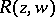, can be considered as single-valued functions of the points on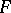.

The integral (1) is then given as the integral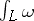of the Abelian differential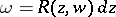ontaken along some rectifiable path. In general, specifying only the initial and end points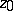andof the path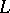does not completely determine the value of the Abelian integral (1), or, which is the same, the integral (1) turns out to be a multi-valued function of the initial and end points of the path.

The behaviour of an Abelian integral ondepends, first of all, on the topological structure of, in particular on the topological invariant called the genus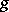of the surface(cf. Genus of a surface). The genusis connected with the number of sheetsand with the number of branch points(counted with multiplicities) by the relation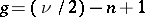. For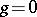the variablesand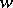can be rationally expressed in some parameter, and the Abelian integral becomes the integral of a rational function in. This will happen, for example, in the elementary cases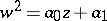and.

If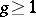, any Abelian integral can be expressed in the form of a linear combination of elementary functions and canonical Abelian integrals of the three kinds. The integralis called an Abelian integral of the first kind ifis an Abelian differential of the first kind. In other words, Abelian integrals of the first kind are characterized by the fact that for a fixed initial pointof the paththey are a function of the upper boundthat is an everywhere finite, usually multi-valued, function on. Such a characterization may be used, for example, to construct analogues of Abelian integrals of the first kind on non-compact Riemann surfaces. Any Abelian integral of the first kind can be represented in the form of a linear combination oflinearly-independent normal Abelian integrals of the first kindof differentialsthat constitute a canonical basis for the Abelian differentials of the first kind. If the surfaceis cut along the cycles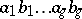of a canonical basis for the homology, a simply-connected domain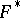is obtained. The integrals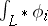are single-valued functions of the upper boundfor all pathswith fixed initial pointand fixed end point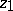. The multi-valuedness of the integrals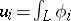along an arbitrary path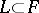joiningwithis now completely described by the fact that it differs from the integralsonly by an integral linear combination of the-periodsand the-periods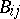of a basis of the differentials of the first kind. These constitute the period matrix of dimension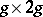, which satisfies the bilinear Riemann relations (cf. Abelian differential).

An integralwhereis an Abelian differential of the second kind is said to be an Abelian integral of the second kind. Considered as a function of the upper bound, it has no singularities anywhere onexcept for poles. An Abelian integral of a normalized Abelian differential of the second kind is known as a normal Abelian integral of the second kind.

An Abelian integral of the third kind is an arbitrary Abelian integral. It usually has logarithmic singularities on; however, such singularities can only occur in pairs. An Abelian integral of a normal Abelian differential of the third kind is called a normal Abelian integral of the third kind. Any Abelian integral can be represented as a linear combination of normal Abelian integrals of the first, second and third kinds. Unlike Abelian integrals of the first and second kinds, Abelian integrals of the third kind usually also have the so-called polar periods, beside the- and-periods (which are called cyclic periods). Polar periods are taken along cycles which are homologous to zero, but encircle the logarithmic singularities of the Abelian integral. They are caused by the poles of the Abelian differentialwith non-zero residues.

A number of relations depending on the topological and conformal structure ofexist for arbitrary Abelian integrals on the same Riemann surface. Thus, ifis a normal Abelian differential of the third kind with simple poles in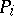and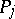, then the following theorem on the permutation of the parameters and the bounds of an Abelian integral of the third kind holds for all points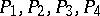: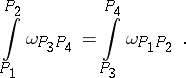Relations which connect Abelian integrals with rational functions onare known as Abelian theorems. In terms of divisors, for example, the Abelian theorem for Abelian integrals of the first kind has the following form: A divisoronis the divisor of a meromorphic function if and only if there exists a chainwith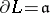and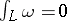for all Abelian differentials of the first kind on. There also exist variants of Abelian theorems for Abelian integrals of the second and third kinds . Abelian integrals and, in particular, Abelian theorems, are the basis of the transcendental construction of the Jacobi variety of a Riemann surface. The question of the inversion of an Abelian integral as a function of its upper bound also leads to the concepts of an Abelian function; an elliptic function; and theta-functions (cf. Theta-function; Jacobi inversion problem).

Historically, the theory of Abelian integrals followed from the consideration of a surface of genus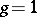. If one writes the corresponding equation in the formwhereis a polynomial inof the third or fourth degree, then one obtains elliptic integrals (cf. Elliptic integral) as the respective Abelian integrals. They first appeared at the end of the 17th century and the beginning of the 18th century as the result of the rectification of curves of the second order in the studies of Jacob and Johann Bernoulli and of G. Fagnano. L. Euler tackled the addition theorem of elliptic integrals, which is a special case of a theorem of N.H. Abel (1752). Abel and C.G.J. Jacobi (1827) stated the problem of inversion of elliptic integrals and obtained the solution. The beginnings of the theory of elliptic functions were thus established. However, some facts concerning this theory had been established by C.F. Gauss early in the 18th century. Abel and Jacobi dealt with the much more difficult case of inversion of Abelian integrals in the case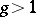. During the very first stages of development stress was laid on hyper-elliptic integrals, wherewith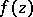a polynomial of the fifth or sixth degree without multiple roots. Here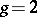and the difficulty of the inversion problem can already be noticed. The principal advances in the theory of inversion of Abelian integrals are due to B. Riemann (1851), who introduced the concept of Riemann surfaces and formulated and gave proofs of a large number of important results.

Multi-dimensional generalizations of the theory of Abelian integrals form the subject matter of algebraic geometry and the theory of complex manifolds.

How to Cite This Entry:
Abelian integral. Encyclopedia of Mathematics. URL: http://encyclopediaofmath.org/index.php?title=Abelian_integral&oldid=13186
This article was adapted from an original article by E.D. Solomentsev (originator), which appeared in Encyclopedia of Mathematics - ISBN 1402006098. See original article